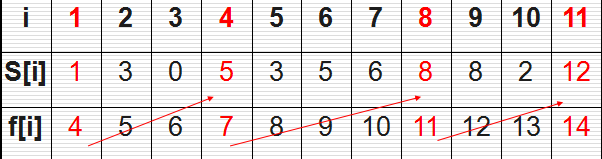### 活動選擇問題理解貪心演算法

{a3,a9,a11}是一個兼容的活動子集，但它不是最大子集，因為子集{a1,a4,a8,a11}更大，實際上它是我們這個問題的最大兼容子集，但它不是唯一的一個{a2，a4，a9，a11}1.動態規劃演算法

```       static void Main(string[] args)
{
//添加兩個活動，一個從0點到0點，一個從24點到24點
//記錄活動start時間的數組
int[] s = { 0, 1, 3, 0, 5, 3, 5, 6, 8, 8, 2, 12, 24 };
//記錄活動end時間的數組
int[] e = { 0, 4, 5, 6, 7, 8, 9, 10, 11, 12, 13, 14, 24 };
//記錄所有方案的二維數組
//數組的行數和列數對應了活動方案的編號，如result[3,7]代表了第3個到第7個活動的活動最大兼容子集
List<int>[,] result = new List<int>[13, 13];
//為二維數組賦初值，集合中的數字代表哪些活動組合成最大兼容子集
for (int m = 0; m < 13; m++)
{
for (int n = 0; n < 13; n++)
{
result[m, n] = new List<int>();
}
}
//雙重循環
//依次遍歷存儲所有活動的最大兼容子集，j是最後一個活動編號，i是第一個活動編號
for (int j = 0; j < 13; j++)
{
for (int i = 0; i < j - 1; i++)
{
//int集合sij用於存儲計算出的第i到第j個活動的最大兼容子集
List<int> sij = new List<int>();
//循環遍歷每一個活動，number是當前指向的活動編號
for (int number = 1; number < s.Length - 1; number++)
{
//如果當前遍歷到的活動的時間在最後一個活動j的開始時間和第一個活動i的結束時間之間，這個活動就能插入兩個活動之間進行
if (s[number] >= e[i] && e[number] <= s[j])
{
sij.Add(number);
}
}
//如果有活動插入到了兩個活動之間
if (sij.Count > 0)
{
//保存最大兼容子集的活動的數量
int maxCount = 0;
//保存最大兼容子集
List<int> tempList = new List<int>();
//循環遍歷插入的活動
foreach (int number in sij)
{
//計算最大兼容子集的活動的數量
int count = result[i, number].Count + result[number, j].Count + 1;
//更新最大兼容子集的活動數量
//可能有多個活動可以插入兩個活動之間，因此需要判斷哪一個活動插入後是從i活動到j活動的最大兼容子集
//將最大兼容子集的活動編號保存起來
if (maxCount < count)
{
maxCount = count;
tempList = result[i, number].Union<int>(result[number, j]).ToList<int>();
tempList.Add(number);
}
}
//更新計算出的i活動到j活動的最大兼容子集
result[i, j] = tempList;
}
}
}
//結果取出第0個活動到第12個活動的最大兼容子集即可
List<int> a = result[0, 12];
foreach (int item in a)
{
Console.Write(item + " ");
}
Console.ReadKey();
}```

2.貪心演算法（遞歸解決）

```        static void Main(string[] args)
{
List<int> list = ActivitySelection(1, 11, 0, 24);
foreach(int t in list)
{
Console.Write(t + " ");
}
Console.ReadKey();
}
static int[] s = { 0, 1, 3, 0, 5, 3, 5, 6, 8, 8, 2, 12, 24 };
static int[] e = { 0, 4, 5, 6, 7, 8, 9, 10, 11, 12, 13, 14, 24 };
/// <summary>
/// 計算給定的活動編號之間的活動能否在給定時間內進行
/// </summary>
/// <param name="startActivityNumber">開始的活動編號</param>
/// <param name="endActivityNumber">結束的活動編號</param>
/// <param name="startTime">開始時間</param>
/// <param name="endTime">結束時間</param>
/// <returns></returns>
public static List<int> ActivitySelection(int startActivityNumber,int endActivityNumber,int startTime,int endTime)
{
//如果活動已經找完或者時間已經找完，結束遞歸調用
if (startActivityNumber > endActivityNumber || startTime >= endTime)
return new List<int>();
//記錄可以插入的活動編號
int tempNumber = 0;
//循環遍歷活動編號，看能否在給定時間內進行
for (int number = startActivityNumber; number <= endActivityNumber; number++)
{
//判斷能否在給定時間內進行
if(s[number] >= startTime && e[number] <= endTime)
{
tempNumber = number;
break;
}
}
//找到了一個能插入的活動後，繼續遞歸找下一個能插入的活動
List<int> list = ActivitySelection(tempNumber + 1, endActivityNumber, e[tempNumber], endTime);
//將找到的活動編號添加入集合中
list.Add(tempNumber);
//返回找到的結果
return list;
}```

3.貪心演算法（迭代解決）

```        static void Main(string[] args)
{
List<int> list = ActivitySelection(1, 11, 0, 24);
foreach(int t in list)
{
Console.Write(t + " ");
}
Console.ReadKey();
}
static int[] s = { 0, 1, 3, 0, 5, 3, 5, 6, 8, 8, 2, 12, 24 };
static int[] e = { 0, 4, 5, 6, 7, 8, 9, 10, 11, 12, 13, 14, 24 };
/// <summary>
/// 計算給定的活動編號之間的活動能否在給定時間內進行
/// </summary>
/// <param name="startActivityNumber">開始的活動編號</param>
/// <param name="endActivityNumber">結束的活動編號</param>
/// <param name="startTime">開始時間</param>
/// <param name="endTime">結束時間</param>
/// <returns></returns>
public static List<int> ActivitySelection(int startActivityNumber,int endActivityNumber,int startTime,int endTime)
{
//保存結果的list
List<int> list = new List<int>();
//循環遍歷活動編號，看能否在給定時間內進行
for (int number = 1; number <= 11; number++)
{
//判斷能否在給定時間內進行，找到可以在給定時間內進行的活動後將活動編號加入結果的集合，並且下一個活動的開始時間需要更新
if(s[number] >= startTime && e[number] <= endTime)
{
list.Add(number);
startTime = e[number];
}
}
//返回找到的結果
return list;
}```